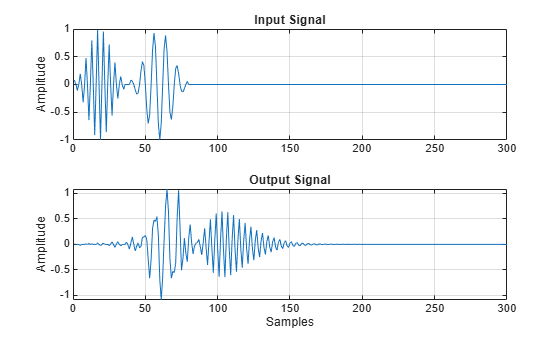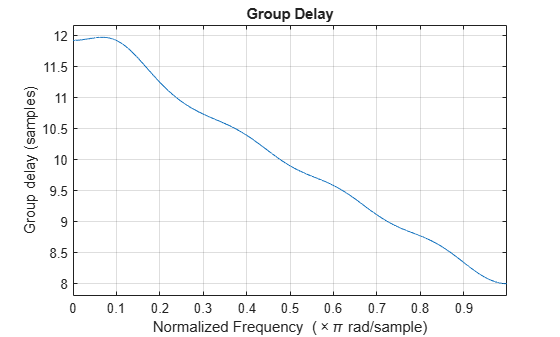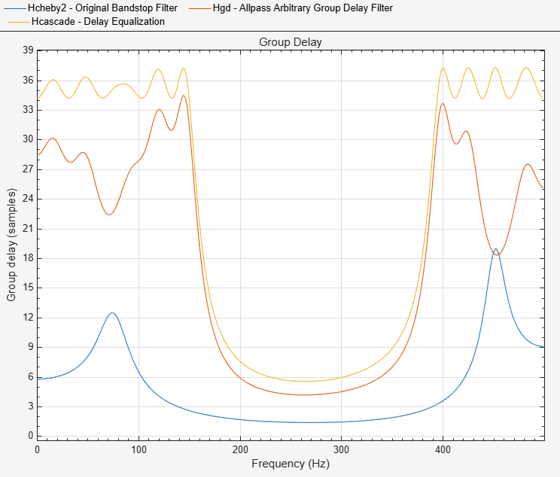Main Content

# fdesign.arbgrpdelay

Arbitrary group delay filter specification object

## Syntax

``` D = fdesign.arbgrpdelay(SPEC) D = fdesign.arbgrpdelay(SPEC,SPEC1,SPEC2,...) D = fdesign.arbgrpdelay(N,F,Gd) D = fdesign.arbgrpdelay(...,Fs) ```

## Description

Arbitrary group delay filters are allpass filters you can use for correcting phase distortion introduced by other filters. `fdesign.arbgrpdelay` uses an iterative least p-th norm optimization procedure to minimize the phase response error .

` D = fdesign.arbgrpdelay(SPEC)` specifies an allpass arbitrary group delay filter with the `Specification` property set to `SPEC`. See Input Arguments for a description of supported specifications.

`D = fdesign.arbgrpdelay(SPEC,SPEC1,SPEC2,...)` initializes the allpass arbitrary group delay filter specification object with specifications `SPEC1,SPEC2,...`. See SPEC for a description of supported specifications.

`D = fdesign.arbgrpdelay(N,F,Gd)` specifies an allpass arbitrary group delay filter. The filter order is equal to `N`, frequency vector equal to `F`, and group delay vector equal to `Gd`. See SPEC for a description of the filter order, frequency vector, and group delay vector inputs. See the example Design an Allpass Filter With an Arbitrary Group Delay for an example using this syntax.

`D = fdesign.arbgrpdelay(...,Fs)` specifies the sampling frequency in hertz as a trailing scalar. If you do not specify a sampling frequency, all frequencies are normalized frequencies and group delay values are in samples. If you specify a sampling frequency, group delay values are in seconds.

## Tips

If your arbitrary group delay design produces the error ```Poorly conditioned Hessian matrix```, attempt one or more of the following:

## Input Arguments

 `SPEC` Filter specification. `SPEC` is one of the following two options. The entries are not case sensitive. `'N,F,Gd'``'N,B,F,Gd'` The filter specifications are defined as follows: `N` — Filter order. This value must be an even positive integer. The numerator and denominator orders are both equal to `N`. Allpass Systems explains why the numerator and denominator filter orders are equal and the order must be even in `fdesign.arbgrpdelay`.`F` — Frequency vector for the group delay specifications. The elements of the frequency vector must increase monotonically. If you do not specify a sampling frequency, `Fs`, in hertz, the frequencies are normalized frequencies. For a single-band design, the first element of the normalized frequency vector must be zero and the last element must be 1. These correspond to 0 and π radians/sample respectively. For multiband designs, the union of the frequency vectors must range from [0,1].If you specify a sampling frequency, `Fs`, the first element of the frequency vector in a single-band design must be 0. The last element must be the Nyquist frequency, Fs/2. For multiband designs, the union of the frequency vectors must range from [0,Fs/2].`Gd` — Group delay vector. A vector with nonnegative elements equal in length to the frequency vector, `F`. The elements of `Gd` specify the nonnegative group delay at the corresponding element of the frequency vector, `F`.If you do not specify a sampling frequency, `Fs`, in Hertz, the group delays are in samples. If you specify a sampling frequency, the group delays are in seconds.`B` — Number of frequency bands. If you use this specification, you must specify a frequency and group delay vector for each band. The union of the frequency vectors must range from [0,1] in normalized frequency, or [0,Fs/2] when a sampling frequency is specified. The elements in the union of the frequency bands must be monotonically increasing.For example:```filtorder = 14; freqband1 = [0 0.1 0.4]; grpdelay1 = [1 2 3]; freqband2 = [0.5 0.8 1]; grpdelay2 = [3 2 1]; D = fdesign.arbgrpdelay('N,B,F,Gd',filtorder,2,freqband1,grpdelay1,freqband2,grpdelay2);``` Default: `'N,F,Gd'` `Fs` Sampling frequency. Specify the sampling frequency as a trailing positive scalar after all other input arguments. Specifying a sampling frequency forces the group delay units to be in seconds. If you specify a sampling frequency, the first element of the frequency vector must be 0. The last element must be the Nyquist frequency, Fs/2.

## Output Arguments

 `D` Filter specification object. An allpass arbitrary group delay filter specification object containing the following modifiable properties: `Specification`, `NormalizedFrequency`, `FilterOrder`, `Frequencies`, and `GroupDelay`. Use the `normalizefreq` method to change the `NormalizedFrequency` property after construction.

## Examples

collapse all

Construct a signal consisting of two discrete-time windowed sinusoids (wave packets) with disjoint time support to illustrate frequency dispersion. One discrete-time sinusoid has a frequency of pi/2 radians/sample and the other has a frequency of pi/4 radians/sample. There are 9 periods of the higher-frequency sinusoid that precede 5 periods of the lower-frequency signal.

Create the signal.

```x = zeros(300,1); x(1:36) = cos(pi/2*(0:35)).*hamming(36)'; x(40:40+39) = cos(pi/4*(0:39)).*hamming(40)';```

Create an arbitrary group delay filter that delays the higher-frequency wave packet by approximately 100 samples.

```N = 18; f = 0:.1:1; gd = ones(size(f));```

Delay pi/2 radians/sample by 100 samples

```gd(6) = 100; d = fdesign.arbgrpdelay(N,f,gd); Hd = design(d,'iirlpnorm','MaxPoleRadius',0.9,'SystemObject',true);```

Visualize the group delay

`fvtool(Hd,'analysis','grpdelay');`Filter the input signal with the arbitrary group delay filter and illustrate the frequency dispersion. The high-frequency wave packet, which initially preceded the low-frequency wave packet, now occurs later because of the nonconstant group delay.

```y = Hd(x); subplot(211) plot(x); title('Input Signal'); grid on; ylabel('Amplitude'); subplot(212); plot(y); title('Output Signal'); grid on; xlabel('Samples'); ylabel('Amplitude');`````` N = 10; f = [0 0.02 0.04 0.06 0.08 0.1 0.25 0.5 0.75 1]; g = [5 5 5 5 5 5 4 3 2 1]; w = [2 2 2 2 2 2 1 1 1 1]; hgd = fdesign.arbgrpdelay(N,f,g); Hgd = design(hgd,'iirlpnorm','Weights',w,'MaxPoleRadius',0.95,... 'SystemObject',true); fvtool(Hgd,'Analysis','grpdelay') ;```Perform multiband delay equalization outside the stopband.

```Fs = 1e3; Hcheby2 = design(fdesign.bandstop('N,Fst1,Fst2,Ast',10,150,400,60,Fs),'cheby2',... 'SystemObject',true); f1 = 0.0:0.5:150; % Hz g1 = grpdelay(Hcheby2,f1,Fs).'/Fs; % seconds f2 = 400:0.5:500; % Hz g2 = grpdelay(Hcheby2,f2,Fs).'/Fs; % seconds maxg = max([g1 g2]); % Design an arbitrary group delay allpass filter to equalize the group % delay of the bandstop filter. Use an order 18 multiband design and specify % two bands. hgd = fdesign.arbgrpdelay('N,B,F,Gd',18,2,f1,maxg-g1,f2,maxg-g2,Fs); Hgd = design(hgd,'iirlpnorm','MaxPoleRadius',0.95,'SystemObject',true); Hcascade = cascade(Hcheby2,Hgd); hft = fvtool(Hcheby2,Hgd,Hcascade,'Analysis','grpdelay','Fs',Fs); legend(hft,'Original Bandstop Filter','Allpass Arbitrary Group Delay Filter',... 'Delay Equalization', 'Location','North');```## More About

collapse all

### Group Delay in Discrete-Time Filter Design

The frequency response of a rational discrete-time filter is:

`$H\left({e}^{j\omega }\right)=\frac{B\left({e}^{j\omega }\right)}{A\left({e}^{j\omega }\right)}$`

The argument of the frequency response as a function of the angle, ω, is referred to as the phase response.

The negative of the first derivative of the argument with respect to ω is the group delay.

`$\tau \left(\omega \right)=-\frac{d}{d\omega }Arg\left(H\left({e}^{j\omega }\right)\right)$`

Systems with nonlinear phase responses have nonconstant group delay, which causes dispersion of the frequency components of the signal. You may not want this phase distortion even if the magnitude distortion introduced by the filter produces the desired effect. See Design an Arbitrary Group Delay Filter for an illustration of frequency dispersion resulting from nonconstant group delay.

In these cases, you can cascade the frequency-selective filter with an allpass filter that compensates for the group delay. This process is often referred to as delay equalization.

### Allpass Systems

The general form of an allpass system function with a real-valued impulse response is:

`${H}_{ap}\left(z\right)=\prod _{k=1}^{M}\frac{{z}^{-1}-{d}_{k}}{1-{d}_{k}{z}^{-1}}\prod _{k=1}^{N}\frac{\left({z}^{-1}-{c}_{k}\right)\left({z}^{-1}-{c}_{k}^{*}\right)}{\left(1-{c}_{k}{z}^{-1}\right)\left(1-{c}_{k}^{*}{z}^{-1}\right)}$`

where the dk denote the real-valued poles and the ck denote the complex-valued poles, which occur in conjugate pairs.

The preceding equation demonstrates that allpass systems with real-valued impulse responses have 2N+M zeros and poles. The poles and zeros occur in pairs with reciprocal magnitudes. The filter order is always the same for the numerator and denominator.

Because `fdesign.arbgrpdelay` uses robust second-order section (biquad) filter structures to implement the allpass arbitrary group delay filter, the filter order must be even.

## Algorithms

`fdesign.arbgrpdelay` uses a least p-th norm iterative optimization described in .

## Alternatives

`iirgrpdelay` — Returns an allpass arbitrary group delay filter. The `iirgrpdelay` function returns the numerator and denominator coefficients. This behavior differs from that of `fdesign.arbgrpdelay`, which returns the filter in second-order sections. `iirgrpdelay` accepts only normalized frequencies.

## References

 Antoniou, A. Digital Signal Processing: Signals, Systems, and Filters., New York:McGraw-Hill, 2006, pp. 719–771.

## See Also

Introduced in R2011b

## Support